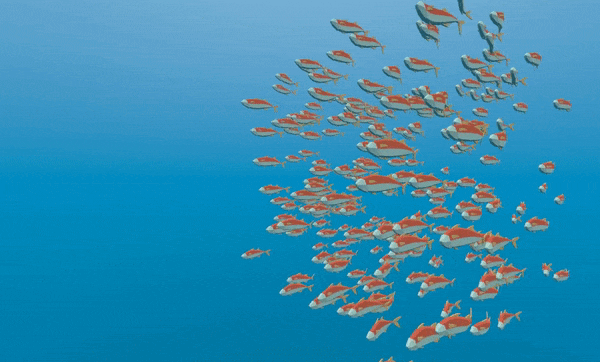# Controlling thousands of fish with Particles¶

The problem with MeshInstances is that it is expensive to update their transform array. It is great for placing many static objects around the scene. But it is still difficult to move the objects around the scene.

To make each instance move in an interesting way we will use a Particles node. Particles take advantage of GPU acceleration by computing and setting the per-instance information in a Shader.

Informacja

Particles are not available in GLES2, instead use CPUParticles, which do the same thing as Particles, but do not benefit from GPU acceleration.

First create a Particles node. Then, under „Draw Passes” set the Particle’s „Draw Pass 1” to your Mesh. Then under „Process Material” create a new ShaderMaterial.

Set the `shader_type` to `particles`.

```shader_type particles
```

Then add the following two functions:

```float rand_from_seed(in uint seed) {
int k;
int s = int(seed);
if (s == 0)
s = 305420679;
k = s / 127773;
s = 16807 * (s - k * 127773) - 2836 * k;
if (s < 0)
s += 2147483647;
seed = uint(s);
return float(seed % uint(65536)) / 65535.0;
}

uint hash(uint x) {
x = ((x >> uint(16)) ^ x) * uint(73244475);
x = ((x >> uint(16)) ^ x) * uint(73244475);
x = (x >> uint(16)) ^ x;
return x;
}
```

These functions come from the default ParticlesMaterial. They are used to generate a random number from each particle’s `RANDOM_SEED`.

A unique thing about particle shaders is that some of the built-in variables are saved across frames. `TRANSFORM`, `COLOR`, and `CUSTOM` can all be accessed in the Spatial shader of the mesh, and also in the particle shader the next time it is run.

Next, setup your `vertex` function. Particles shaders only contain a vertex function and no others.

First we will distinguish between code that needs to be run only when the particle system starts and code that should always run. We want to give each fish a random position and a random animation offset when the system is first run so we wrap that code in an `if` statement that checks the built-in variable `RESTART` which becomes `true` for one frame when the particle system is restarted.

From a high level, this looks like:

```void vertex() {
if (RESTART) {
//Initialization code goes here
} else {
//per-frame code goes here
}
}
```

Next, we need to generate 4 random numbers: 3 to create a random position and one for the random offset of the swim cycle.

First, generate 4 seeds inside the `RESTART` block using the `hash` function provided above:

```uint alt_seed1 = hash(NUMBER + uint(1) + RANDOM_SEED);
uint alt_seed2 = hash(NUMBER + uint(27) + RANDOM_SEED);
uint alt_seed3 = hash(NUMBER + uint(43) + RANDOM_SEED);
uint alt_seed4 = hash(NUMBER + uint(111) + RANDOM_SEED);
```

Then, use those seeds to generate random numbers using `rand_from_seed`:

```CUSTOM.x = rand_from_seed(alt_seed1);
vec3 position = vec3(rand_from_seed(alt_seed2) * 2.0 - 1.0,
rand_from_seed(alt_seed3) * 2.0 - 1.0,
rand_from_seed(alt_seed4) * 2.0 - 1.0);
```

Finally, assign `position` to `TRANSFORM.xyz`, which is the part of the transform that holds the position information.

```TRANSFORM.xyz = position * 20.0;
```

Remember, all this code so far goes inside the `RESTART` block.

The vertex shader for your mesh can stay the exact same as it was in the previous tutorial.

Now you can move each fish individually each frame, either by adding to the `TRANSFORM` directly or by writing to `VELOCITY`.

Let’s transform the fish by setting their `VELOCITY`.

```VELOCITY.z = 10.0;
```

This is the most basic way to set `VELOCITY` every particle (or fish) will have the same velocity.

Just by setting `VELOCITY` you can make the fish swim however you want. For example, try the code below.

```VELOCITY.z = cos(TIME + CUSTOM.x * 6.28) * 4.0 + 6.0;
```

This will give each fish a unique speed between `2` and `10`.

If you used `CUSTOM.y` in the last tutorial, you can also set the speed of the swim animation based on the `VELOCITY`. Just use `CUSTOM.y`.

```CUSTOM.y = VELOCITY.z * 0.1;
```

This code gives you the following behavior:Using a ParticlesMaterial you can make the fish behavior as simple or complex as you like. In this tutorial we only set Velocity, but in your own Shaders you can also set `COLOR`, rotation, scale (through `TRANSFORM`). Please refer to the Particles Shader Reference for more information on particle shaders.